﻿

### Almost conformal 2-cosymplectic pseudo-Sasakian manifolds

#### Abstract

In the last years several papers have been concerned with almost r-contact or r-paracontact manifolds (see  and ). On the other hand, V.V. Goldberg and R. Rosta have recently studied in  almost 1-contact pseudo-Riemannian manifolds which are endowed with a conformal cosymplectic pseudo-Sasakian structure. Since the manifolds M which we are going to discuss are connected and paracompact,we denote by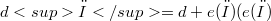$dω=d+e(ω) (e(ω)$: exterior product by the closed 1-form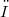$ω$ ) the cohomology operator (see ) on M. Then any form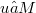$u∈ M$ such that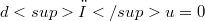$dω u=0$ is said to be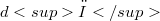$dω$-closed. The present paper is devoted to the study of even dimensional pseudo Riemannian manifolds of signature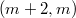$(m + 2,m)$ which are endowed with an almost conformal 2-cosymplectic pseudo-Sasakian structure. Such a manifold is denoted by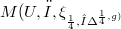$M(U, ω, \xi_𝛼, η^𝛼,g)$, and its structure tensor fields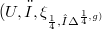$(U,ω,\xi_𝛼,η^𝛼,g)$ are: the paracomplex operator (see ), an exterior recurrent (see ) 2-form of rank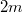$2m$, two structure vector fields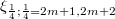$\xi_𝛼; 𝛼=2m+1, 2m+2$, two structure 1-forms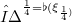$η^𝛼=\flat(\xi_𝛼)$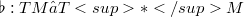$\flat: TM→ T* M$ is the musical isomorphism  defined by g) and the pseudo-Riemannian tensor g of M respectively.

DOI Code: 10.1285/i15900932v8n1p123

Full Text: PDF